# 2070: Trig Identities

 Trig Identities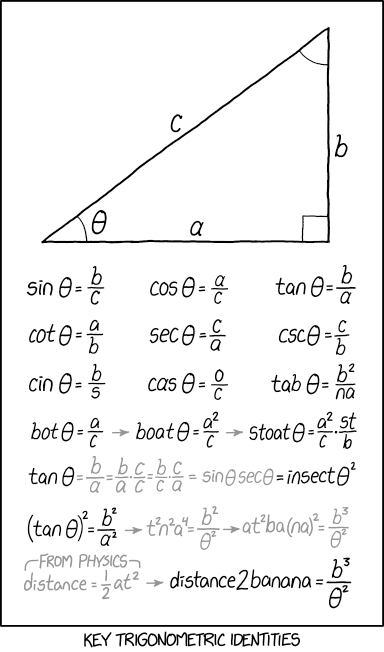Title text: ARCTANGENT THETA = ENCHANT AT TARGET

## Explanation

This comic shows several real trigonometric identities at the first two lines and further below some identities "derived" by applying algebraic methods to the letters in the trigonometric function names, which is obviously nonsense.

The first line are the known trigonometric functions: sine, cosine and tangent, and the second line contains the reciprocals of the trigonometric functions from the first line: cosecant, secant, and cotangent.

The following identities are made up and are increasing in absurdity. The comic reflects on the confusion one gets when working more intensely with these identities, since there are a lot of hidden dependencies between them. You can also check how they are related through the various Trigonometry Formulas.

The third and fourth line is made by treating the trigonometric function as a product of variables rather than a function and then using the above identities to create words. e.g. sin = b/c -> cin = b/s (this could also be a reference to the C++ cin).

The second to last line performs some algebra on the individual letters of (tan θ)² = b²/a² as a setup to the last line. The last line takes the formula distance = 1/2 a​t² "from physics" and plugs it into the equation of the previous line, doing some algebra to replace a​t² with distance2 and expanding (na)² into nana to get the final equation, distance2banana = b³/θ². This is valid algebra only if the trigonometric operators are taken as variable products rather than operators, but this is a common misconception encountered when people first learn trigonometry. The distance equation is the distance a constantly accelerating object initially at rest moves in a given length of time t, most often used to find how far an object dropped from rest will fall under the influence of gravity in a given amount of time (or how long it will take to fall a given distance).

There are a few formulas that have mistakes if you simply make algebraic manipulations to the six standard trigonometric functions.

• cas θ = o/c seems to be derived from cos θ = a/c but to reach "cas" from "cos" one has to divide by "o" and multiply by "a". This would lead to cas θ = a²/o​c.
• In the identity sin θ sec θ = insect θ² one of the "s"'s has turned into a "t", however this may be reached by 'phonetic stretch' from the sound of saying 'sin sec' together being similar to the sound of the word "insect". Another possible conversion is if you treat "s" as seconds, then "t" could be time, which keeps with the identity theme.

The title text is an anagram. Due to the commutative property of multiplication (which states that order does not affect the product), these equations are equivalent if treated as individual variables as earlier. Another layer of absurdity is added in that the variable Theta is spelled out and broken into its letters, which are then treated as individual variables. (The arctangent referred to here is the inverse tangent, a one-sided inverse to the tangent function. You would not normally write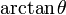$\arctan\theta$, since the theta in the comic refers to an angle, and the arctangent has an angle as its value rather than as its argument; however, using theta here is merely unconventional, not forbidden.) The arctangent generally produces theta, the meaning of it being taken on theta being poorly understood. Randall here elucidates, via tongue-in-cheek algebraic proof, that taking a second arctangent of theta produces magical effects.

### From physics (and beyond)

The formula s=1/2 a​t² gives the distance a uniform accelerating object reaches over time. The second formula belongs to astronomy and the third law of Kepler in which the square of the orbital period of a planet is directly proportional to the cube of the semi-major axis of its orbit, meaning the fraction of b3 and t2 is a constant (banana).

But using the angle θ as an argument leads to Richard Feynman, who did many famous Lectures on Physics and his lost lecture about the Motion of Planets Around the Sun from 1964 in which he only used geometry, based on the orbital ellipse, a circle around, and matching right-angled triangles to illustrate this law from Kepler. For deeper understanding why it really does work there is a nice presentation at the "Journal of Symbolic Geometry": Feynman Says: “Newton implies Kepler, No Calculus Needed! (Brian Beckman, 2006)”

### Proof of algebraic mistakes in the comic

Some have tried to argue there are mathematical justifications for the errors in some of the formulas, by stating (without proof) that you could prove that valid solutions to the original six trig identities (where letters are taken to be variables multiplied together) can be manipulated to show that solutions must have

a=o and s=t.

These proofs are incorrect and can be shown easily with a counterexample. If you make the following assignments of variables like

o=s=1/2 and set c=e=2
while leaving the other variables set to 1 (a=b=i=n=t=θ=1). This variable assignment will simultaneously satisfy all six original trig identities: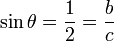$\sin \theta = \frac{1}{2} = \frac{b}{c}$;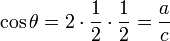$\cos \theta = 2\cdot \frac{1}{2}\cdot\frac{1}{2}=\frac{a}{c}$;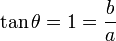$\tan \theta = 1 = \frac{b}{a}$;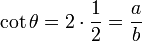$\cot \theta = 2\cdot\frac{1}{2} = \frac{a}{b}$;$\sec \theta = \frac{1}{2}\cdot2\cdot2 = \frac{c}{a}=2$;$\csc \theta = 2\cdot\frac{1}{2}\cdot2 = \frac{c}{b} = 2$.

However in this valid assignment, we have$a\neq o$ since$1 \neq \frac{1}{2}$ and we have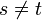$s \neq t$ as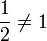$\frac{1}{2} \neq 1$.

This demonstrates that you can not make a valid algebraic derivation of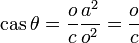$\operatorname{cas} \theta = \frac{o}{c} \frac{a^{2}}{o^2} = \frac{o}{c}$ or$\sin \theta \sec \theta = \operatorname{insect} \theta^{2}$

without additional assumptions beyond the six given trigonometric identities.

## Transcript

[Inside a single frame comic a right-angled triangle is shown. The shorter sides are labeled "a" and "b" and the hypotenuse has a "c". All angles are marked: the right angle by a square and the two others by arcs. One arc (enclosed by "a" and "c") is labeled by the Greek symbol theta (θ).]
[Supposed trigonometric functions of the marked angle θ are shown:]
sin θ = b/c
cos θ = a/c
tan θ = b/a
cot θ = a/b
sec θ = c/a
csc θ = c/b
cin θ = b/s
cas θ = o/c
tab θ = b²/n​a
bot θ = a/c → boat θ = a²/c → stoat θ = a²/c · s​t/b
tan θ ( = b/a = b/a · c/c = b/c · c/a = sin θ sec θ ) = insect θ²
(tan θ)² = b²/a² ( → t²n²a⁴ = b²/θ² → a​t²b​a(n​a)² = b³/θ²
from physics: distance = 1/2 a​t² → ) distance2banana = b³/θ²
[Caption below the frame:]
Key trigonometric identitiesadd a comment! ⋅add a topic (use sparingly)! ⋅refresh comments!

# Discussion

I am confused by the insect line. This seems to be true only if s=t. 141.101.96.209 19:03, 9 November 2018 (UTC)

I added a note regarding how similar it sounds to 'sinsec'. 172.68.51.154 01:47, 10 November 2018 (UTC)
That one and the cas aren't making any sense to me. GreatBigDot (talk) 20:02, 9 November 2018 (UTC)
Oh, the casinus is much important to... What was it? --Dgbrt (talk) 20:15, 9 November 2018 (UTC)
cas is realtively easy... it is cos(theta)=a/c -> cs(theta)=ao/c -> cas(theta)=o/c; when you realise that the top one isn't zero but o it clicks 141.101.96.209 23:35, 9 November 2018 (UTC)
You made the same error Randall did: you divided by 'o' on the left and multiplied on the right. I think the theme of the page is expanding significantly upon common math errors that were already humorous, like the common proof of 5=3 by dividing and multiplying by zero. The error here is in line with the theme of casual beginner errors. 172.68.51.154
You can see cin is derived from sin by swapping the positions of c and s. Likewise, Switching the a and o in cos(theta) = a/c gives cas(theta) = o/c i.e. no need for multiplicative consistency. The rule of treating things as a product of terms is implemented fully in the following lines. 162.158.91.83 11:23, 12 November 2018 (UTC)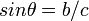$sin \theta = b/c$ leading to$cin \theta = b/s$ is algebraically valid if you interpret sin as the product of s, i, n by multiplying both sides by c/s. It is not valid to just "swap" two letters in one equation that is part of a system of equations. You could do the same trick and get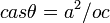$cas \theta = a^2/oc$ from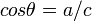$cos \theta = a/c$ or start with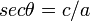$sec \theta = c/a$ and get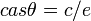$cas \theta = c/e$. Note for all equations except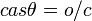$cas \theta = o/c$ and switching an$s$ to a$t$ to find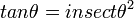$tan \theta = insect \theta^2$, the equations can be correctly derived by treating trig functions as product of single letter variables and algebraically manipulating them. Jimbob (talk) 16:59, 12 November 2018 (UTC)
I think insect is.. a bug.. ;) Smerriman (talk) 20:18, 9 November 2018 (UTC)

Is Enchant at target a magic:the gathering reference? AncientSwordRage (talk) 20:55, 9 November 2018 (UTC)

I think it is a Magic: The Gathering reference. Although it is phrased oddly. You'd think it would be "at target enchantment", rather than "target at enchantment". --Dryhamm (talk) 21:04, 9 November 2018 (UTC)
To me it sounds more like a reference to a nerdy video game, where a certain object worked like this, turning e.g. BEAM OF DARK ENERGY into a BAKED FERRY GNOME 108.162.246.11 21:53, 12 November 2018 (UTC)
That was incredible! (assuming previous poster discovered the extrapolated proof in the description) 172.68.51.154 01:47, 10 November 2018 (UTC)
Combining$\cos\theta=\frac{a}{c}$ and$\mathrm{cas}\ \theta=\frac{o}{c}$ allows you to conclude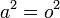$a^2 = o^2$, not$a=o$. 162.158.146.10 (talk) (please sign your comments with ~~~~)

Somebody added a comment on puns, e.g. that "cin sucks". More explanation is needed. It looks like some kind of a meta-joke. If you ask why, and start interpreting, you see that "b/c" == "because". It might be the answer to why the puns line should be removed, though. 172.68.51.154

For the Bot->Boat->Stoat line, this comes from the word game where you add/change letters to make a new word. Start with bot=a/c, multiply by a on both sides gets boat=a^2/c. Multiply by st on both sides and divide b on both sides gets Stoat=a^2/c*St/b. 162.158.78.166 (talk) (please sign your comments with ~~~~)

Checking through the math, just working from the real trig identities, without considering Randall's at-first-glance questionable identities like cas theta = o/c, basically everything that does not have a factor of d or 2 in it is equal to 1, and d is equal to 1/2, which then establishes the more questionable identities as tautological, 1=1. 162.158.142.100 04:09, 10 November 2018 (UTC)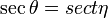$\sec\theta = sect \eta$ 141.101.104.71 13:36, 10 November 2018 (UTC) AndreasH

Am I the only one who saw t²n²a⁴ as "tuna"? 172.68.58.233 14:17, 10 November 2018 (UTC)$\frac{d}{dx}\sec x=\sec x\tan x=$ sex tanks. Probably not Douglas Hofstadter (talk) 21:36, 11 November 2018 (UTC)
From when I saw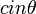$cin\theta$ I knew the rest of the comic would be$\frac{b}{s}$. Observer of the Absurd (talk) 13:28, 15 May 2019 (UTC)